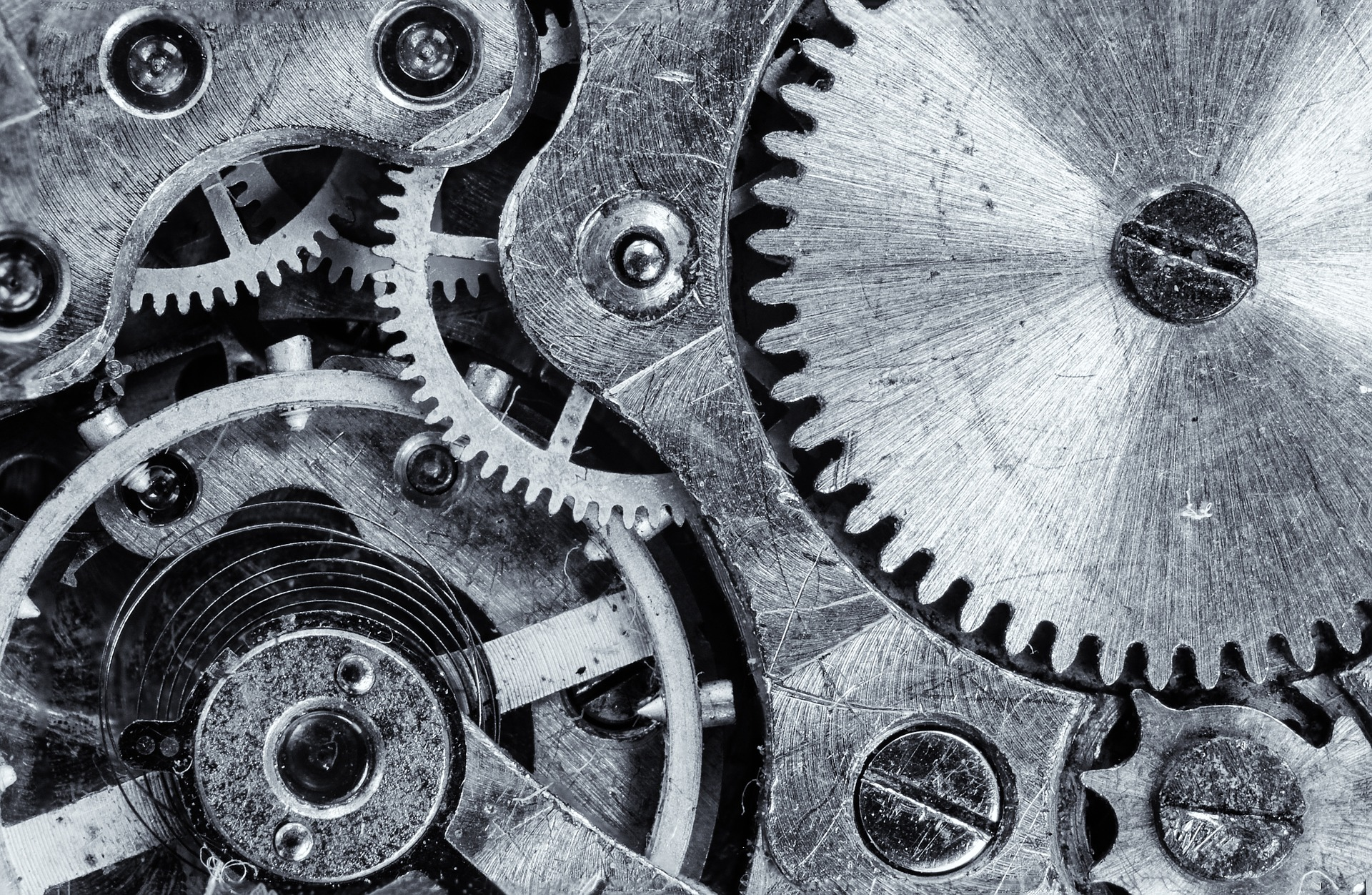## The Importance of Determining Number of Stages in Reciprocating Compressor and Bonus Calculation Spreadsheet

Three previous posts show comparison of number of stages in determining reciprocating compressor power.

Estimate reciprocating compressor power (2-stage compression)

Estimate reciprocating compressor power (3-stage compression)

Estimate reciprocating compressor power (2-stage compression)

Let’s summarize the results. Read More## Centrifugal Compressor Power Calculation

In this post I want to share you how to calculate centrifugal compressor power since there are so many process engineers are looking for it (and I also don’t know how).

To calculate centrifugal compressor power, we need these gas properties: compressibility factor (Z), molecular weight (MW), inlet gas temperature, inlet gas pressure, outlet gas pressure, adiabatic component (Cp/Cv), and mass flow rate.

Let’s start with an example while I show you step-by-step of the calculation method. In this example, we have these gas properties (I use US units).

Compressibility factor (Z) = 0.9972

Molecular weight (MW) = 18.15

Gas constant (R) = 1544/molecular weight

Inlet temperature (T1)  = 546 deg R

Inlet pressure (P1) = 15 psia (don’t forget to use absolute pressure)

Outlet pressure (P2) = 60 psia

K (Cp/Cv) = 1,274

Flow (W) = 0,184 lb/min